# Double strands

The bilateral way of 329 vs 289 of 286 objects of 2nd prime identity will be taken as the basis that is involving 26-9=17 of other identities where the square of 17 which is 17x17=329 acts as the central of syntax algorithm.

``````id: 26

---+-----+-----+-----+-----+
1 |   5 |   1 |  6  |   7 |----------------------------
---+-----+-----+-----+-----+                            |
2 |   2 |   7 |  9  |  16 |----------------------      |
---+-----+-----+-----+-----+                      |     |
3 |  58 |  10 |  68 |  78 |----------------      |     |
---+-----+-----+-----+-----+                |     |     |
4 |  35 |  69 | 104 | 173 |----------      |     |     |
---+-----+-----+-----+-----+          |     |     |     |
5 | {17}| 105 | 122 |{227}|          |     |     |     |
---+-----+-----+-----+-----+- Cross  {17}Δ26|43Δ30|13Δ17|30
6 | {17}|{123}| 140 | 263 |          |     |     |     |
---+-----+-----+-----+-----+          |     |     |     |
7 |  18 | 141 | 159 | 300 |----------      |     |     |
---+-----+-----+-----+-----+                |     |     |
8 |  15 | 160 | 175 | 335 |----------------      |     |
---+-----+-----+-----+-----+                      |     |
9 |  15 | 176 | 191 | 367 |----------------------      |
---+-----+-----+-----+-----+                            |
10 |  35 |{192}|{227}| 419 |----------------------------
---+-----+-----+-----+-----+
``````

By taking the 200 residual objects we will get their recycing scenario which is similar to the leading and lagging strands of DNA Replication where the result is obtained on the lagging stage by initiating a DNA synthesis called RNA Primer.

RNA primers are used by living organisms in initiation of synthesizing a strand of DNA. A class of enzymes called primases add a complementary RNA primer to the reading template on both the leading and lagging strands (Wikipedia).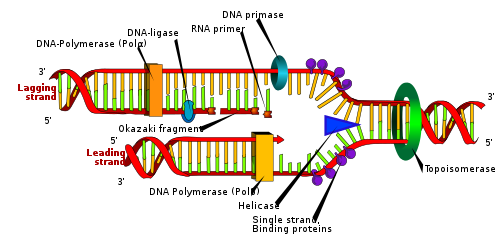There are situations where theories in two or three spacetime dimensions are useful for describing phenomena in condensed matter physics. Finally, there exist scenarios in which there could actually be more than 4D of spacetime.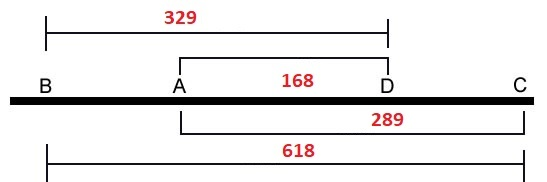Note that 1/109's decimal expansion is period 108 (making it a ‘long period prime' in that 1/p has the maximal period of p−1 digits). This period consists of 54 bilateral 9 sums = 486, which is the number of primes in the 243 pairs summing to 7920.

36 + 72 = 108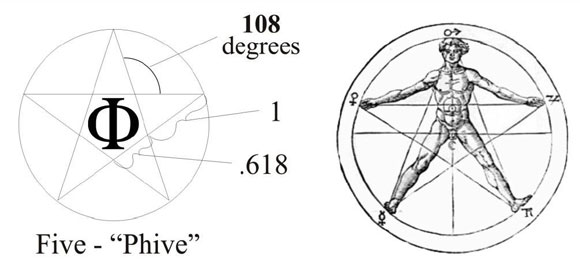This behaviour would come to the feature of golden ratio. However it is not stand as a basic rule but as an impact of 329's vs 289's layers. That is also the reason why we could only see the three (3) digits of 618 out of the Fibonaci constant.

φ = 1.618 = Fibonaci = Golden Ratio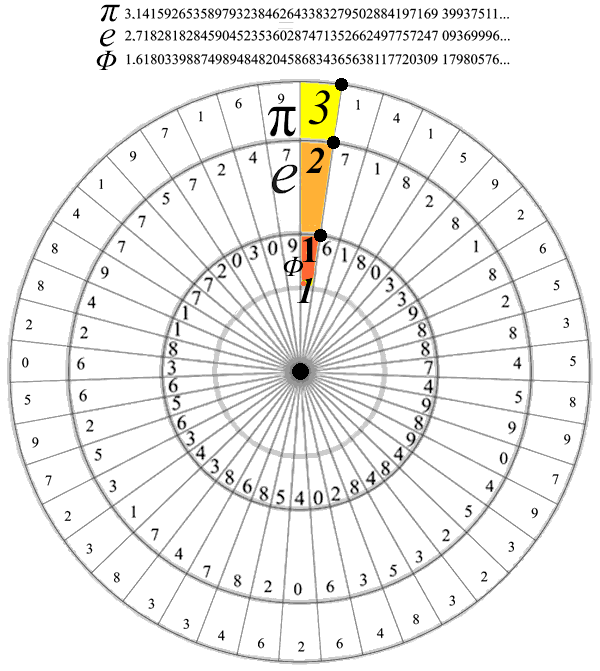The 200 objects that goes from the 2nd to 3rd prime identity would take position as multiplication zone by given of 100x2=200 which is then be used in turn to 100+2=102 (addition zone) and 100^2=10000 (exponentiation zone)

2 + 60 + 40 = 102

``````1st layer:
It has a total of 1000 numbers
Total primes = π(1000) = 168 primes

2nd layer:
It will start by π(168)+1 as the 40th prime
It has 100x100 numbers or π(π(10000)) = 201 primes
Total cum primes = 168 + (201-40) = 168+161 = 329 primes

3rd layer:
Behave the same as 2nd layer which has a total of 329 primes
The primes will start by π(π(π(1000th prime)))+1 as the 40th prime
This 1000 primes will become 1000 numbers by 1st layer of the next level
Total of all primes = 329 + (329-40) = 329+289 = 618 = 619-1 = 619 primes - Δ1
``````

So it is converting all residual objects out of the prime recycling of Riemann Zeta in to those three (3) basic arithmetic operations of Euler's identity as well the Fibonacy constant (φ) to Euler's number (e). Thus none of residual is neglected by an assumption.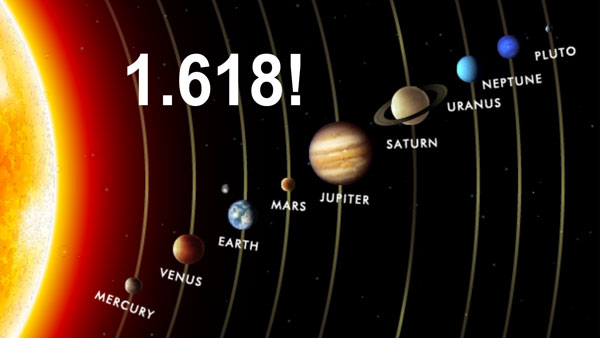DNA polymerase (responsible for DNA replication) enzymes are only capable of adding nucleotides to the 3'-end of an existing nucleic acid, requiring a primer be bound to the template before DNA polymerase can begin a complementary strand.

DNA polymerase adds nucleotides after binding to RNA primer and synthesizes the whole strand. Later, the RNA strands must be removed accurately and replace them with DNA nucleotides forming a gap region known as a nick that is filled in using an enzyme called ligase (Wikipedia).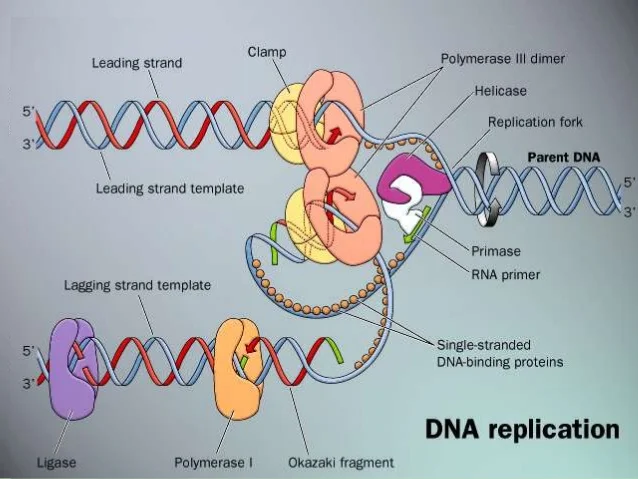Plottng 40th prime scheme of the three (3) layers with all the features of 3rd prime identity with DNA Replication as explained above then they would form their recycing through the three (3) times bilateral 9 sums as shown below.

329 + 109 + 109 + 71 = 329 + 289 = 618 = 1000/1.618 = 1000/φ

``````layer | node | sub |  i      |   f
------+------+-----+---------+------                                             ---
|      |     |  1,2:1 √|  71 (2,3) -------------                            |
|      |  1  +---------+                        |                           |
|  1   |     |      2  |                        |                          (5)
|      |-----+---------+                        |                           |
|      |     |      3  |                        |                           |
1   +------+  2  +---------+----                    |                          ---
|      |     |      4  |                        |                           |
|      +-----+---------+                        |                           |
|  2   |     |      5  |                        |                          (7)
289 √ |      |  3  +---------+                        |                           |
|     |      |     |      6  |                        | {6®}                      |
------+------+-----+---------+------      } (36)      | ↓                        ---
|      |     |      7  |                        | 29=MEC30 - Δ1             |
|      |  4  +---------+                        | ↓                         |
|  3   |     |      8  | 109 (26) √ --          | 9+60+40 from 329-40 √    (11)
|      +-----+---------+              |         | ↓ lagging √               |
|      |     |      9  |  69 (27) √   | {2®}    | 9+60=40+29 from 168 √     |
2   +------|  5  +---------+-----         |         | ↓ leading √              ---
|      |     |      10 | 109 (28) √ --          | 9+60+40 goes to 329 √     |
|      |-----+---------+                        | ↓                         |
|  4   |     |      11 | 71 (29,30,31,32) ------  ↓                        (13)
329 √ |      |  6  +---------+                          9+9+9=27 (Triangular) √   |
|     |      |     |      12 | {70} (36)                                          |
------+------+-----+---------+------------------                                 ---
|      |     |   20:13 |                                                    |
|      |  7  +---------+                                                    |
|  5   |     |      14 |                                                  (17)
|      |-----+---------+                                                    |
|      |     |      15 |                                                    |
3   +------+  8  +---------+-----                                              ---
|      |     |      16 |                                                    |
|      |-----+---------+                                                    |
|  6   |     |      17 | 100(50)                                          (19)
168   |      |  9  +---------+                                                    |
|     |      |     |      18 | 50(68)                                             |
------|------|-----+---------+------                                             ---
``````